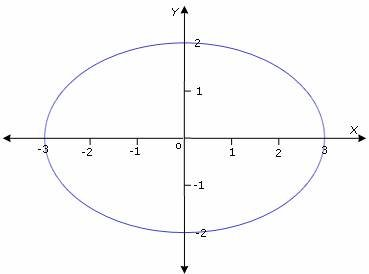Definition Of Ellipse

An Ellipse is a type of conic section that is formed when a cone is cut by a plane.

Major axis is the longest segment extending from one end to the other end on an ellipse and always passes through foci.
Minor axis is the line segment perpendicular to major axis, passing through the center of the ellipse and terminates on the ellipse.
Semi-latus rectum is the distance from focus of the ellipse to ellipse itself.
In an ellipse, the locus of all the points on it is at constant distance from two fixed points, which are called the foci of the ellipse
The figure given represents the major axis, minor axis, semi-latus rectum and foci of the ellipse.

Example of Ellipse

All the planets move around the sun in elliptical orbits.

Solved Example on Ellipse

Ques: Find the x - intercepts of the ellipse shown.Choices:

A. (- 3, 0), (3, 0)
B. (- 1, 0), (1, 0)
C. (- 2, 0), (2, 0)
D. (0, 2), (0, - 2)